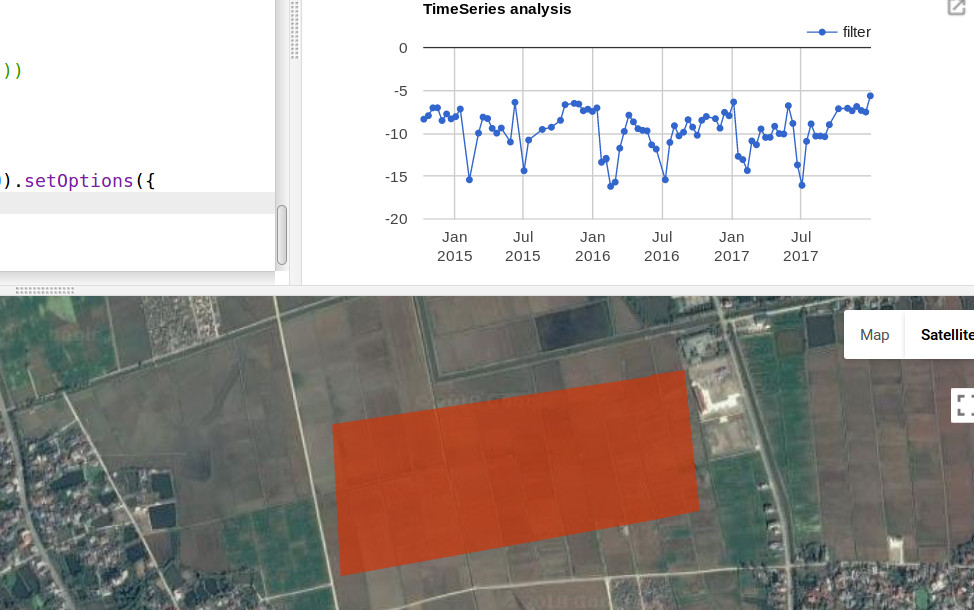# Timeseries with SAR

Check what is underneath the clouds with sentinel 1

With sentinel 1 we  can measure the earth where it’s cloudy or dark. Sentinel 1 uses synthetic aperture radar (SAR), a technology that can capture images in any light or weather condition. below an example to create timeseries with SAR using a speckle filter.  See the example here.

```
// import sentinel 1 and filter data series
var S1 = ee.ImageCollection('COPERNICUS/S1_GRD')
.filter(ee.Filter.listContains('transmitterReceiverPolarisation', 'VH'))
.filter(ee.Filter.listContains('transmitterReceiverPolarisation', 'VV'))
.filter(ee.Filter.eq('instrumentMode', 'IW'))
.filter(ee.Filter.eq('orbitProperties_pass', 'DESCENDING'))
.filterBounds(roi)
.filterDate('2014-01-01','2017-12-31')
.select(["VV","VH"])

//Function to convert from dB
function toNatural(img) {
return ee.Image(10.0).pow(img.select(0).divide(10.0));
}
//Function to convert to dB
function toDB(img) {
return ee.Image(img).log10().multiply(10.0);
}

&nbsp;

//Apllying a Refined Lee Speckle filter as coded in the SNAP 3.0 S1TBX:
//https://github.com/senbox-org/s1tbx/blob/master/s1tbx-op-sar-processing/src/main/java/org/esa/s1tbx/sar/gpf/filtering/SpeckleFilters/RefinedLee.java
//Adapted by Guido Lemoine

function RefinedLee(img) {
// img must be in natural units, i.e. not in dB!
// Set up 3x3 kernels

// convert to natural.. do not apply function on dB!
var myimg = toNatural(img);

var weights3 = ee.List.repeat(ee.List.repeat(1,3),3);
var kernel3 = ee.Kernel.fixed(3,3, weights3, 1, 1, false);

var mean3 = myimg.reduceNeighborhood(ee.Reducer.mean(), kernel3);
var variance3 = myimg.reduceNeighborhood(ee.Reducer.variance(), kernel3);

// Use a sample of the 3x3 windows inside a 7x7 windows to determine gradients and directions
var sample_weights = ee.List([[0,0,0,0,0,0,0], [0,1,0,1,0,1,0],[0,0,0,0,0,0,0], [0,1,0,1,0,1,0], [0,0,0,0,0,0,0], [0,1,0,1,0,1,0],[0,0,0,0,0,0,0]]);

var sample_kernel = ee.Kernel.fixed(7,7, sample_weights, 3,3, false);

// Calculate mean and variance for the sampled windows and store as 9 bands
var sample_mean = mean3.neighborhoodToBands(sample_kernel);
var sample_var = variance3.neighborhoodToBands(sample_kernel);

// Determine the 4 gradients for the sampled windows
var gradients = sample_mean.select(1).subtract(sample_mean.select(7)).abs();
gradients = gradients.addBands(sample_mean.select(6).subtract(sample_mean.select(2)).abs());
gradients = gradients.addBands(sample_mean.select(3).subtract(sample_mean.select(5)).abs());
gradients = gradients.addBands(sample_mean.select(0).subtract(sample_mean.select(8)).abs());

// And find the maximum gradient amongst gradient bands
var max_gradient = gradients.reduce(ee.Reducer.max());

// Create a mask for band pixels that are the maximum gradient
var gradmask = gradients.eq(max_gradient);

// duplicate gradmask bands: each gradient represents 2 directions
gradmask = gradmask.addBands(gradmask);

// Determine the 8 directions
var directions = sample_mean.select(1).subtract(sample_mean.select(4)).gt(sample_mean.select(4).subtract(sample_mean.select(7))).multiply(1);
directions = directions.addBands(sample_mean.select(6).subtract(sample_mean.select(4)).gt(sample_mean.select(4).subtract(sample_mean.select(2))).multiply(2));
directions = directions.addBands(sample_mean.select(3).subtract(sample_mean.select(4)).gt(sample_mean.select(4).subtract(sample_mean.select(5))).multiply(3));
directions = directions.addBands(sample_mean.select(0).subtract(sample_mean.select(4)).gt(sample_mean.select(4).subtract(sample_mean.select(8))).multiply(4));
// The next 4 are the not() of the previous 4
directions = directions.addBands(directions.select(0).not().multiply(5));
directions = directions.addBands(directions.select(1).not().multiply(6));
directions = directions.addBands(directions.select(2).not().multiply(7));
directions = directions.addBands(directions.select(3).not().multiply(8));

// Mask all values that are not 1-8
directions = directions.updateMask(gradmask);

// "collapse" the stack into a singe band image (due to masking, each pixel has just one value (1-8) in it's directional band, and is otherwise masked)
directions = directions.reduce(ee.Reducer.sum());

var sample_stats = sample_var.divide(sample_mean.multiply(sample_mean));

// Calculate localNoiseVariance
var sigmaV = sample_stats.toArray().arraySort().arraySlice(0,0,5).arrayReduce(ee.Reducer.mean(), );

// Set up the 7*7 kernels for directional statistics
var rect_weights = ee.List.repeat(ee.List.repeat(0,7),3).cat(ee.List.repeat(ee.List.repeat(1,7),4));

var diag_weights = ee.List([[1,0,0,0,0,0,0], [1,1,0,0,0,0,0], [1,1,1,0,0,0,0],
[1,1,1,1,0,0,0], [1,1,1,1,1,0,0], [1,1,1,1,1,1,0], [1,1,1,1,1,1,1]]);

var rect_kernel = ee.Kernel.fixed(7,7, rect_weights, 3, 3, false);
var diag_kernel = ee.Kernel.fixed(7,7, diag_weights, 3, 3, false);

// Create stacks for mean and variance using the original kernels. Mask with relevant direction.
var dir_mean = myimg.reduceNeighborhood(ee.Reducer.mean(), rect_kernel).updateMask(directions.eq(1));
var dir_var = myimg.reduceNeighborhood(ee.Reducer.variance(), rect_kernel).updateMask(directions.eq(1));

dir_mean = dir_mean.addBands(myimg.reduceNeighborhood(ee.Reducer.mean(), diag_kernel).updateMask(directions.eq(2)));
dir_var = dir_var.addBands(myimg.reduceNeighborhood(ee.Reducer.variance(), diag_kernel).updateMask(directions.eq(2)));

// and add the bands for rotated kernels
for (var i=1; i&lt;4; i++) {
dir_mean = dir_mean.addBands(myimg.reduceNeighborhood(ee.Reducer.mean(), rect_kernel.rotate(i)).updateMask(directions.eq(2*i+1)));
dir_var = dir_var.addBands(myimg.reduceNeighborhood(ee.Reducer.variance(), rect_kernel.rotate(i)).updateMask(directions.eq(2*i+1)));
dir_mean = dir_mean.addBands(myimg.reduceNeighborhood(ee.Reducer.mean(), diag_kernel.rotate(i)).updateMask(directions.eq(2*i+2)));
dir_var = dir_var.addBands(myimg.reduceNeighborhood(ee.Reducer.variance(), diag_kernel.rotate(i)).updateMask(directions.eq(2*i+2)));
}

// &quot;collapse&quot; the stack into a single band image (due to masking, each pixel has just one value in it&#039;s directional band, and is otherwise masked)
dir_mean = dir_mean.reduce(ee.Reducer.sum());
dir_var = dir_var.reduce(ee.Reducer.sum());

// A finally generate the filtered value
var varX = dir_var.subtract(dir_mean.multiply(dir_mean).multiply(sigmaV)).divide(sigmaV.add(1.0));

var b = varX.divide(dir_var);

var result = dir_mean.add(b.multiply(myimg.subtract(dir_mean)));
//return(result);
return(img.addBands(ee.Image(toDB(result.arrayGet(0))).rename(&quot;filter&quot;)));
}

var collection = S1.map(RefinedLee);
var col = ee.ImageCollection(collection.select(&quot;filter&quot;));

print(ui.Chart.image.series(col, roi, ee.Reducer.mean(), 20).setOptions({
title: &#039;TimeSeries analysis&#039;,
lineWidth: 1,
pointSize: 3 }));

```

## 7 comments

1.SOUMYA KANTA DAS says:

can you please explain what it really does. doe it measures the change in the surface in time series

2.dassoumya says:

Can u explain what it does. Does it measure change in surface during the timeseries

3.Thomas says:

Hi,
what’s the variable ‘filter’ used for the chart? which polarisation does it comes from?

4.Bacgiang says:

how is ‘filter’ calculated?

5.thisearthsite says:

it is the output from the RefinedLee function

6.venkata sai krishna vanama says:

when I run this speckle filter in google colab the following error has occurred.

directions = directions.addBands(directions.select(0).not().multiply(5));
File “”, line 39
directions = directions.addBands(directions.select(0).not().multiply(5));
^
SyntaxError: invalid syntax

1.thisearthsite says:

check you .not function